# Quick Review: Coordinate Geometry

In Coordinate Geometry, we use graphs and coordinates to find measurements of geometric figures.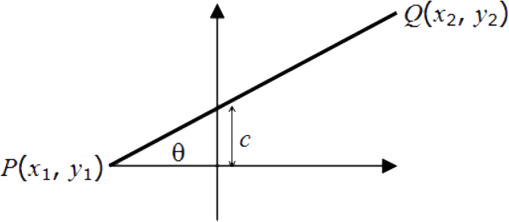###### Important Formulas
• Distance PQ =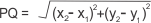• Slope of PQ = m =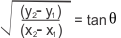• Equation of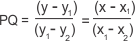or y = mx + c
• The product of the slopes of two perpendicular lines is –1.
• The distance between the points (x1, y1) and (x2, y2) is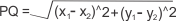• If point P(x, y) divides the segment AB, where A ≡ (x1, y1) and B ≡ (x2, y2), internally in the ratio m: n, then,
x= (mx2 + nx1)/(m+n)
and
y= (my2 + ny1)/(m+n)
• If P is the midpoint then,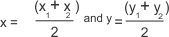• If G (x, y) is the centroid of triangle ABC, A ≡ (x1, y1), B ≡ (x2, y2), C ≡ (x3, y3), then,
x = (x1 + x2 + x3)/3 and y = (y1 + y2 + y3)/3

• If I (x, y) is the in-centre of triangle ABC, A ≡ (x1, y1), B ≡ (x2, y2), C ≡ (x3, y3), then,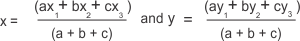where a, b and c are the lengths of the BC, AC and AB respectively.
• The equation of a straight line is y = mx + c, where m is the slope and c is the y-intercept (tan θ = m, where θ is the angle that the line makes with the positive X-axis).